CGAL 5.5 - Point Set Processing
User Manual

# Introduction

This CGAL component implements methods to analyze and process 3D point sets. The input is an unorganized 3D point set, possibly with normal attributes (unoriented or oriented). The input point set can be analyzed to measure geometric properties such as average spacing between the points and their k nearest neighbors. It can be processed with functions devoted to the simplification, regularization, upsampling, outlier removal, smoothing, normal estimation and normal orientation. The processing of point sets is often needed in applications dealing with measurement data, such as surface reconstruction from laser scanned data (see Figure 79.1).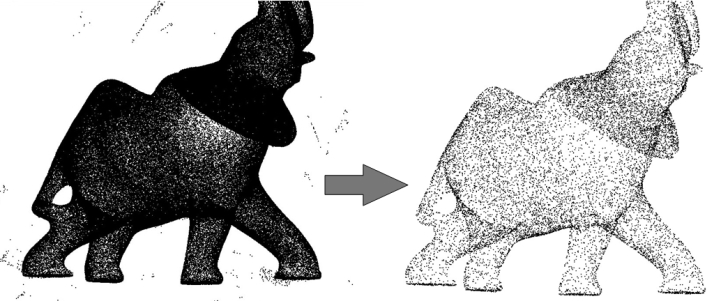Figure 79.1 Point set processing. Left: 275K points sampled on the statue of an elephant with a Minolta laser scanner. Right: point set after outlier removal, denoising and simplification to 17K points.

In the context of surface reconstruction we can position the elements of this component along the common surface reconstruction pipeline (Figure 79.2) which involves the following steps:

1. Scanning and scan registration to produce a set of points or points with normals;
2. Outlier removal;
3. Simplification to reduce the number of input points;
4. Smoothing to reduce noise in the input data;
5. Normal estimation and orientation when the normals are not already provided by the acquisition device; and
6. Surface reconstruction. Chapter Poisson Surface Reconstruction deals with surface reconstruction from point sets with normal attributes.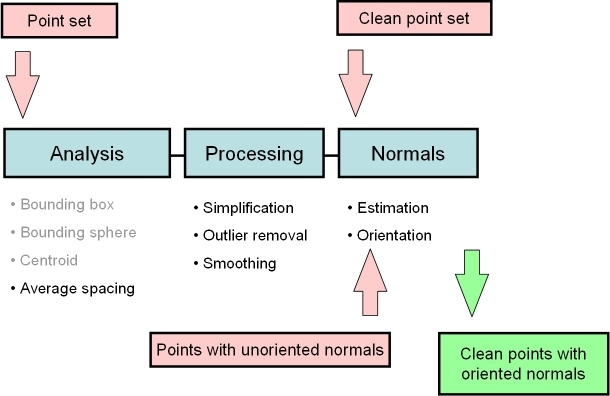Figure 79.2 Point set processing pipeline for surface reconstruction. The algorithms listed in gray are available from other CGAL components (bounding volumes and principal component analysis).

# API

The algorithms of this component take as input parameters ranges of 3D points, or of 3D points with normals. They can be adapted to the user's data structures and make extensive use of named parameters and of property maps:

std::vector<PointVectorPair> points;
<CGAL::Parallel_tag> // concurrency tag
(points, // input range of points
12, // parameter: number of neighbors
CGAL::parameters:: // named parameters:
point_map (Point_map()). // * point map
normal_map (Vector_map())); // * normal map

This API was introduced in CGAL 4.12. Please refer to the dedicated section on how to upgrade from the outdated API.

## Named Parameters

Named parameters are used to deal with optional parameters. The page Named Parameters explains the rationale and API in general. The page Named Parameters describes their usage.

## Property Maps

The property maps are used to access the point or normal information from the input data, so as to let the user decide upon the implementation of a point with normal. The latter can be represented as, e.g., a class derived from the CGAL 3D point, or as a std::pair<Point_3<K>, Vector_3<K>>, or as a boost::tuple<..,Point_3<K>, ..., Vector_3<K> >.

The following classes described in Chapter CGAL and Boost Property Maps provide property maps for the implementations of points with normals listed above:

• Identity_property_map<T>
• First_of_pair_property_map<Pair> and Second_of_pair_property_map<Pair>
• Nth_of_tuple_property_map<N, Tuple>

Identity_property_map<Point_3> is the default value of the position property map expected by all functions in this component.

See below examples using pair and tuple property maps.

Users of this package may use other types to represent positions and normals if they implement the corresponding property maps.

Points and normals can even be stored in separate containers and accessed by their index, as any built-in vector is also a property map.

#include <CGAL/Exact_predicates_inexact_constructions_kernel.h>
#include <CGAL/grid_simplify_point_set.h>
#include <CGAL/property_map.h>
#include <vector>
#include <fstream>
// types
typedef Kernel::Point_3 Point;
typedef Kernel::Vector_3 Vector;
int main(int argc, char*argv[])
{
const std::string fname = (argc>1) ? argv : CGAL::data_file_path("points_3/fin90_with_PCA_normals.xyz");
// Reads a .xyz point set file in points[].
std::vector<Point> points;
std::vector<Vector> normals;
std::ifstream stream(fname);
Point p;
Vector v;
while(stream >> p >> v)
{
points.push_back(p);
normals.push_back(v);
}
std::cout << points.size() << " input points" << std::endl;
std::vector<std::size_t> indices(points.size());
for(std::size_t i = 0; i < points.size(); ++i){
indices[i] = i;
}
// simplification by clustering using erase-remove idiom
double cell_size = 0.05;
std::vector<std::size_t>::iterator end;
cell_size,
CGAL::parameters::point_map (CGAL::make_property_map(points)));
std::size_t k = end - indices.begin();
std::cerr << "Keep " << k << " of " << indices.size() << " indices" << std::endl;
{
std::vector<Point> tmp_points(k);
std::vector<Vector> tmp_normals(k);
for(std::size_t i=0; i<k; ++i){
tmp_points[i] = points[indices[i]];
tmp_normals[i] = normals[indices[i]];
}
points.swap(tmp_points);
normals.swap(tmp_normals);
}
std::cout << points.size() << " points after the simplification" << std::endl;
return EXIT_SUCCESS;
}

## Upgrading from pre-CGAL 4.12 API

The current API based on ranges and named parameters was introduced in CGAL 4.12. The old API that used pairs of iterators along with usual C++ parameters (with some default values and overloads to handle optional parameters) has been removed in CGAL 5.0.

Translating your pre-CGAL 4.12 code using Point Set Processing to the current API is easy. For example, consider this code using the old API:

std::vector<PointVectorPair> points;
// Old pre-CGAL 4.12 API
CGAL::jet_estimate_normals<Concurrency_tag>
(points.begin(), points.end(),
12); // Number of neighbors

The pair of iterators is replaced by a range and the optional parameters (than can be deduced automatically in simple cases) are moved to the end of the function in a single named parameter object (see Named Parameters). The code translated to the current API becomes:

std::vector<PointVectorPair> points;
// New API
CGAL::jet_estimate_normals<Concurrency_tag>
(points,
12, // Number of neighbors
CGAL::parameters::point_map (CGAL::First_of_pair_property_map<PointVectorPair>()).

Please refer to the Reference Manual for the detailed API of the Point Set Processing functions.

# Input/Output

## Points And Normals

CGAL provides functions to read and write sets of points (possibly with normals) from the following file formats:

All of the functions in I/O Functions (with the exception of the LAS format) can read and write either points alone or points with normals (depending on whether the normal_map named parameter is used by the user or not). Note that the PLY format handles both ASCII and binary formats. In addition, PLY and LAS are extensible formats that can embed additional properties. These can also be read by CGAL (see Section Points With Properties).

### Example

The following example reads a point set from an input file and writes it to a file, both in the XYZ format. Positions and normals are stored in pairs and accessed through property maps.

#include <CGAL/Exact_predicates_inexact_constructions_kernel.h>
#include <CGAL/property_map.h>
#include <CGAL/IO/write_xyz_points.h>
#include <utility> // defines std::pair
#include <vector>
#include <fstream>
#include <iostream>
// types
typedef Kernel::Point_3 Point;
typedef Kernel::Vector_3 Vector;
// Point with normal vector stored as a std::pair.
typedef std::pair<Point, Vector> Pwn;
int main(int argc, char*argv[])
{
const std::string fname = (argc>1) ? argv : CGAL::data_file_path("points_3/oni.pwn");
// Reads a .xyz point set file in points[].
// Note: read_points() requires an output iterator
// over points and as well as property maps to access each
// point position and normal.
std::vector<Pwn> points;
std::back_inserter(points),
CGAL::parameters::point_map(CGAL::First_of_pair_property_map<Pwn>())
{
std::cerr << "Error: cannot read file " << fname << std::endl;
return EXIT_FAILURE;
}
// Saves point set.
// Note: write_XYZ() requires property maps to access each
// point position and normal.
if(!CGAL::IO::write_XYZ("oni_copy.xyz", points,
CGAL::parameters::point_map(CGAL::First_of_pair_property_map<Pwn>())
.stream_precision(17)))
return EXIT_FAILURE;
return EXIT_SUCCESS;
}

## Points With Properties

PLY files are designed to embed an arbitrary number of additional attributes. More specifically, point sets may contain visibility vectors, RGB colors, intensity, etc. As it is not possible to provide dedicated functions to every possible combination of PLY properties, CGAL provides a simple way to read PLY properties and store them in any structure the user needs. Handling of LAS files works similarly with the difference that the property names and types are fixed and defined by the LAS standard.

Functions read_PLY_with_properties() and read_LAS_with_properties() allow the user to read any property needed. The user must provide a set of property handlers that are used to instantiate number types and complex objects from PLY/LAS properties. This handlers are either:

• a pair consisting of a property map and of a single PLY/LAS property descriptor
• a tuple consisting of a property map, a functor to construct the objects wanted and multiple PLY/LAS property descriptors

Output functions write_PLY_with_properties() and write_LAS_with_properties() work similarly.

### PLY Writing Example

The following example shows how to call write_PLY_with_properties() to write a point set with points, RGB colors and intensity. Notice that in order to write a complex object, users need to provide an overload of CGAL::Output_rep.

#include <CGAL/Exact_predicates_inexact_constructions_kernel.h>
#include <CGAL/property_map.h>
#include <CGAL/IO/write_ply_points.h>
#include <utility>
#include <vector>
#include <fstream>
// types
typedef Kernel::FT FT;
typedef Kernel::Point_3 Point;
typedef Kernel::Vector_3 Vector;
typedef std::array<unsigned char, 4> Color;
// Point with normal, color and intensity
typedef std::tuple<Point, Color, int> PCI;
// Define how a color should be stored
namespace CGAL {
template< class F >
struct Output_rep< ::Color, F > {
const ::Color& c;
static const bool is_specialized = true;
Output_rep (const ::Color& c) : c(c)
{ }
std::ostream& operator() (std::ostream& out) const
{
if (IO::is_ascii(out))
out << int(c) << " " << int(c) << " " << int(c) << " " << int(c);
else
out.write(reinterpret_cast<const char*>(&c), sizeof(c));
return out;
}
};
} // namespace CGAL
int main(int, char**)
{
std::vector<PCI> points; // store points
for (int i = 0; i < 10; ++ i)
points.push_back (std::make_tuple (Point (i / 10., i / 20., i / 30.),
CGAL::make_array (static_cast<unsigned char>(255 / (i + 1)),
static_cast<unsigned char>(192 / (i + 1)),
static_cast<unsigned char>(128 / (i + 1)),
static_cast<unsigned char>(64 / (i + 1))),
i));
std::ofstream f("out.ply", std::ios::binary);
CGAL::IO::set_binary_mode(f); // The PLY file will be written in the binary format
std::make_tuple(Color_map(),
std::make_pair(Intensity_map(), CGAL::IO::PLY_property<int>("intensity")));
return EXIT_SUCCESS;
}

The following example shows how to call read_PLY_with_properties() to read a point set with points, normals, RGB colors and intensity and stores these attributes in a user-defined container.

#include <CGAL/Exact_predicates_inexact_constructions_kernel.h>
#include <CGAL/property_map.h>
#include <utility>
#include <vector>
#include <fstream>
// types
typedef Kernel::FT FT;
typedef Kernel::Point_3 Point;
typedef Kernel::Vector_3 Vector;
typedef std::array<unsigned char, 3> Color;
// Point with normal, color and intensity
typedef std::tuple<Point, Vector, Color, int> PNCI;
int main(int argc, char*argv[])
{
const std::string fname = (argc>1) ? argv : CGAL::data_file_path("points_3/colors.ply");
// Reads a .ply point set file with normal vectors and colors
std::vector<PNCI> points; // store points
std::ifstream in(fname);
std::make_pair(Intensity_map(), CGAL::PLY_property<int>("intensity")),
std::make_tuple(Color_map(),
{
std::cerr << "Error: cannot read file " << fname << std::endl;
return EXIT_FAILURE;
}
for(std::size_t i = 0; i < points.size (); ++ i)
{
const Point& p = get<0>(points[i]);
const Vector& n = get<1>(points[i]);
const Color& c = get<2>(points[i]);
int I = get<3>(points[i]);
std::cerr << "Point (" << p
<< ") with normal (" << n
<< "), color (" << int(c) << " " << int(c) << " " << int(c)
<< ") and intensity " << I << std::endl;
}
return EXIT_SUCCESS;
}

The following example shows how to call read_LAS_with_properties() to read a point set with points and RGBI colors and stores these attributes in a user-defined container.

#include <CGAL/Exact_predicates_inexact_constructions_kernel.h>
#include <CGAL/property_map.h>
#include <utility>
#include <vector>
#include <fstream>
// types
typedef Kernel::FT FT;
typedef Kernel::Point_3 Point;
typedef std::array<unsigned short, 4> Color;
typedef std::pair<Point, Color> PointWithColor;
int main(int argc, char*argv[])
{
const char* fname = (argc>1) ? argv : "data/pig_points.las";
// Reads a .las point set file with normal vectors and colors
std::ifstream in(fname, std::ios_base::binary);
std::vector<PointWithColor> points; // store points
CGAL::IO::LAS_property::R(),
CGAL::IO::LAS_property::G(),
CGAL::IO::LAS_property::B(),
CGAL::IO::LAS_property::I())))
{
std::cerr << "Error: cannot read file " << fname << std::endl;
return EXIT_FAILURE;
}
for(std::size_t i = 0; i < points.size(); ++ i)
std::cout << points[i].first << std::endl;
return EXIT_SUCCESS;
}
Point Set IO

# Average Spacing

Function compute_average_spacing() computes the average spacing of all input points to their k nearest neighbor points, k being specified by the user. As it provides an order of a point set density, this function is used downstream the surface reconstruction pipeline to automatically determine some parameters such as output mesh sizing for surface reconstruction.

## Example

The following example reads a point set in the xyz format and computes the average spacing. Index, position and color are stored in a tuple and accessed through property maps.
File Point_set_processing_3/average_spacing_example.cpp

#include <CGAL/Exact_predicates_inexact_constructions_kernel.h>
#include <CGAL/compute_average_spacing.h>
#include <vector>
#include <fstream>
#include <boost/tuple/tuple.hpp>
// Types
typedef Kernel::FT FT;
typedef Kernel::Point_3 Point;
// Data type := index, followed by the point, followed by three integers that
// define the Red Green Blue color of the point.
typedef boost::tuple<int, Point, int, int, int> IndexedPointWithColorTuple;
// Concurrency
typedef CGAL::Parallel_if_available_tag Concurrency_tag;
int main(int argc, char*argv[])
{
const std::string fname = (argc>1)?argv:CGAL::data_file_path("points_3/sphere_20k.xyz");
// Reads a file in points.
// As the point is the second element of the tuple (that is with index 1)
// we use a property map that accesses the 1st element of the tuple.
std::vector<IndexedPointWithColorTuple> points;
{
std::cerr << "Error: cannot read file " << fname << std::endl;
return EXIT_FAILURE;
}
// Initialize index and RGB color fields in tuple.
// As the index and RGB color are respectively the first and third-fifth elements
// of the tuple we use a get function from the property map that accesses the 0
// and 2-4th elements of the tuple.
for(unsigned int i = 0; i < points.size(); i++)
{
points[i].get<0>() = i; // set index value of tuple to i
points[i].get<2>() = 0; // set RGB color to black
points[i].get<3>() = 0;
points[i].get<4>() = 0;
}
// Computes average spacing.
const unsigned int nb_neighbors = 6; // 1 ring
FT average_spacing = CGAL::compute_average_spacing<Concurrency_tag>(
points, nb_neighbors,
std::cout << "Average spacing: " << average_spacing << std::endl;
return EXIT_SUCCESS;
}

Note that other functions such as centroid or bounding volumes are found in other CGAL components:

• centroid()
• bounding_box()
• Min_sphere_of_spheres_d<Traits>

# Automatic Scale Estimation

Point sets are often used to sample objects with a higher dimension, typically a curve in 2D or a surface in 3D. In such cases, finding the scale of the objet is crucial, that is to say finding the minimal number of points (or the minimal local range) such that the subset of points has the appearance of a curve in 2D or a surface in 3D .

CGAL provides two functions that automatically estimate the scale of a 2D point set sampling a curve or a 3D point set sampling a surface:

• estimate_global_k_neighbor_scale()
• estimate_global_range_scale()

Functions such as grid_simplify_point_set() require a range scale while jet_estimate_normals(), remove_outliers() or vcm_estimate_normals() are examples of functions that accepts both a K neighbor scale or a range scale.

In some specific cases, the scale of a point set might not be homogeneous (for example if the point set contains variable noise). CGAL also provides two functions that automatically estimate the scales of a point set at a set of user-defined query points:

• estimate_local_k_neighbor_scales()
• estimate_local_range_scales()

The 4 functions presented here work both with 2D points and 3D points and they shouldn't be used if the point sets do not sample a curve in 2D or a surface in 3D.

## Global Scale Example

The following example reads a 3D point set in the xyz format and:

• Estimates the K neighbor global scale
• Uses it to smooth the point set
• Estimates the range global scale
• Uses it to simplify the point set
#include <CGAL/Exact_predicates_inexact_constructions_kernel.h>
#include <CGAL/estimate_scale.h>
#include <CGAL/jet_smooth_point_set.h>
#include <CGAL/grid_simplify_point_set.h>
#include <CGAL/Timer.h>
#include <CGAL/Memory_sizer.h>
#include <vector>
#include <fstream>
// Concurrency
typedef CGAL::Parallel_if_available_tag Concurrency_tag;
// Types
typedef Kernel::FT FT;
typedef Kernel::Point_3 Point_3;
int main (int argc, char** argv)
{
const std::string fname = (argc>1)?argv:CGAL::data_file_path("points_3/sphere_20k.xyz");
std::vector<Point_3> points;
{
std::cerr << "Error: can't read input file" << std::endl;
return EXIT_FAILURE;
}
// estimate k scale
std::size_t k_scale = CGAL::estimate_global_k_neighbor_scale (points);
// Example: use estimated k as scale for jet smoothing
CGAL::jet_smooth_point_set<Concurrency_tag>(points, static_cast<unsigned int>(k_scale));
// estimate range scale
FT range_scale = CGAL::estimate_global_range_scale (points);
// Example: use estimated range for grid simplification
points.erase(CGAL::grid_simplify_point_set(points, range_scale), points.end());
// print some informations on runtime
std::size_t memory = CGAL::Memory_sizer().virtual_size();
std::cout << "Scales computed in " << time << " second(s) using "
<< (memory>>20) << " MiB of memory:" << std::endl;
std::cout << " * Global K scale: " << k_scale << std::endl;
std::cout << " * Global range scale: " << range_scale << std::endl;
return EXIT_SUCCESS;
}

## Local Scales Example

This second example generates a 2D point set sampling a circle with variable noise. It then estimates the scale at 3 different query points in the domain.

#include <CGAL/Exact_predicates_inexact_constructions_kernel.h>
#include <CGAL/estimate_scale.h>
#include <CGAL/Random.h>
#include <vector>
#include <fstream>
// Types
typedef Kernel::FT FT;
typedef Kernel::Point_2 Point_2;
int main (int, char**)
{
std::vector<Point_2> samples;
samples.reserve (100000);
// Generate circle with gradually variable noise
// - noise-free for points with x close to (-1)
// - noisy for points with x close to (+1)
for (std::size_t i = 0; i < 100000; ++ i)
{
FT theta = CGAL::get_default_random().get_double(0, 2. * CGAL_PI);
FT noise = 0.5 * (std::cos(theta) + 1.) * CGAL::get_default_random().get_double(0., 0.2);
int mult = (CGAL::get_default_random().get_bool() ? 1 : -1);
samples.push_back (Point_2 (std::cos(theta) * (1. + mult * noise * noise),
std::sin(theta) * (1. + mult * noise * noise)));
}
// Search for local scales on 3 different locations
std::vector<Point_2> queries;
queries.reserve (3);
queries.push_back (Point_2 (-1., 0.));
queries.push_back (Point_2 (0., 1.));
queries.push_back (Point_2 (1., 0.));
std::vector<std::size_t> k_scales;
queries,
std::back_inserter (k_scales));
// Display results
std::cerr << "K-Scales found:" << std::endl
<< " - On noise-free region: " << k_scales << std::endl // Should be small
<< " - On moderately noisy region: " << k_scales << std::endl // Should be higher
<< " - On very noisy region: " << k_scales << std::endl; // Should be even higher
return EXIT_SUCCESS;
}

# Registration

CGAL provides two functions as wrapper for the OpenGR library , and two functions as wrapper for the PointMatcher library :

• CGAL::OpenGR::compute_registration_transformation() computes the registration of one point set w.r.t. another in the form of a CGAL::Aff_transformation_3 object, using the Super4PCS algorithm ;
• CGAL::OpenGR::register_point_sets() computes the registration of one point set w.r.t. another and directly aligns it to it;
• CGAL::pointmatcher::compute_registration_transformation() computes the registration of one point set w.r.t. another in the form of a CGAL::Aff_transformation_3 object, using the ICP (Iterative Closest Point) algorithm;
• CGAL::pointmatcher::register_point_sets() computes the registration of one point set w.r.t. another and directly aligns it to it.

## OpenGR Example

The following example reads two point sets and aligns them using the OpenGR library, using the Super4PCS algorithm:
File Point_set_processing_3/registration_with_OpenGR.cpp

#include <CGAL/Simple_cartesian.h>
#include <CGAL/IO/write_points.h>
#include <CGAL/property_map.h>
#include <CGAL/OpenGR/compute_registration_transformation.h>
#include <CGAL/OpenGR/register_point_sets.h>
#include <fstream>
#include <iostream>
#include <utility>
typedef K::Point_3 Point_3;
typedef K::Vector_3 Vector_3;
typedef std::pair<Point_3, Vector_3> Pwn;
namespace params = CGAL::parameters;
int main(int argc, const char** argv)
{
const std::string fname1 = (argc>1) ? argv : CGAL::data_file_path("points_3/hippo1.ply");
const std::string fname2 = (argc>2) ? argv : CGAL::data_file_path("points_3/hippo2.ply");
std::vector<Pwn> pwns1, pwns2;
CGAL::parameters::point_map(CGAL::First_of_pair_property_map<Pwn>())
.normal_map(Normal_map())))
{
std::cerr << "Error: cannot read file " << fname1 << std::endl;
return EXIT_FAILURE;
}
CGAL::parameters::point_map(Point_map())
.normal_map(Normal_map())))
{
std::cerr << "Error: cannot read file " << fname2 << std::endl;
return EXIT_FAILURE;
}
// EITHER call the registration method Super4PCS from OpenGR to get the transformation to apply to pwns2
// std::pair<K::Aff_transformation_3, double> res =
params::point_map(Point_map())
.normal_map(Normal_map())
.number_of_samples(200)
.maximum_running_time(60)
.accuracy(0.01),
params::point_map(Point_map())
.normal_map(Normal_map()));
// OR call the registration method Super4PCS from OpenGR and apply the transformation to pwn2
double score =
params::point_map(Point_map())
.normal_map(Normal_map())
.number_of_samples(200)
.maximum_running_time(60)
.accuracy(0.01),
params::point_map(Point_map())
.normal_map(Normal_map()));
if(!CGAL::IO::write_points("pwns2_aligned.ply", pwns2,
CGAL::parameters::point_map(Point_map())
.normal_map(Normal_map())
.stream_precision(17)))
return EXIT_FAILURE;
std::cout << "Registration score: " << score << ".\n"
<< "Transformed version of " << fname2
<< " written to pwn2_aligned.ply.\n";
return EXIT_SUCCESS;
}

Figure 79.4 demonstrates visualization of a scan data before and after different registration methods are applied, including the OpenGR registration method. To obtain the results for OpenGR registration in the visualization table, above-mentioned example was used.

### Parameter: number_of_samples

Input clouds are sub-sampled prior exploration, to ensure fast computations. Super4PCS has a linear complexity w.r.t. the number of input samples, allowing to use larger values than 4PCS. Simple geometry with large overlap can be matched with only 200 samples. However, with Super4PCS, smaller details can be used during the process by using up to thousands of points. There is no theoretical limit to this parameter; however, using too large values leads to very a large congruent set, which requires more time and memory to be explored.

Using a large number of samples is recommended when:

• geometrical details are required to perform the matching, for instance to disambiguate between several similar configurations,
• the clouds have a very low overlap: using a too sparse sampling can result in an empty overlapping area, causing the algorithm to fail,
• the clouds are very noisy, and require a dense sampling.

Note that Super4PCS is a global registration algorithm, which finds a good approximate of the rigid transformation aligning two clouds. Increasing the number of samples in order to get a fine registration is not optimal: it is usually faster to use fewer samples, and refine the transformation using a local algorithm, like the ICP, or its variant SparseICP.

### Parameter: accuracy

This parameter controls the registration accuracy: setting a small value means that the two clouds need to be very close to be considered as well aligned. It is expressed in scene units.

A simple way to understand its impact is to consider the computation of the Largest Common Pointset (LCP), the metric used to verify how aligned the clouds are. For each transformation matrix produced by Super4PCS, OpenGR computes the LCP measure by considering a shell around the reference cloud, and count the percentage of points of the target cloud lying in the shell. The thickness of the shell is defined by the parameter delta (accuracy).

Using too wide values will slow down the algorithm by increasing the size of the congruent set, while using to small values prevents to find a solution. This parameter impacts other steps of the algorithm, see the paper  for more details.

### Parameter: maximum normal deviation

This parameter sets an angle threshold above which two pairs of points are discarded as candidates for matching. It is expressed in degrees.

The default value is 90° (no filtering). Decreasing this value allows to decrease the computation time by being more selective on candidates. Using too small values might result in ignoring candidates that should indeed have been matched and may thus result in a quality decrease.

### Parameter: overlap

Ratio of expected overlap between the two point sets: it is ranging between 0 (no overlap) to 1 (100% overlap).

The overlap parameter controls the size of the basis used for registration, as shown below:Figure 79.3 The effect of varying overlap parameter on the size of the basis used for registration. The overlap is smaller for left (a) than right (b).

Usually, the larger the overlap, the faster the algorithm. When the overlap is unknown, a simple way to set this parameter is to start from 100% overlap, and decrease the value until obtaining a good result. Using too small values will slow down the algorithm, and reduce the accuracy of the result.

### Parameter: maximum_running_time

Maximum number of seconds after which the algorithm stops. Super4PCS explores the transformation space to align the two input clouds. Since the exploration is performed randomly, it is recommended to use a large time value to explore the whole space.

## PointMatcher Example

The following example reads two point sets and aligns them using the PointMatcher library, using the ICP algorithm. It also shows how to customize ICP algorithm by using possible configurations:
File Point_set_processing_3/registration_with_pointmatcher.cpp

#include <CGAL/Simple_cartesian.h>
#include <CGAL/IO/write_points.h>
#include <CGAL/property_map.h>
#include <CGAL/Aff_transformation_3.h>
#include <CGAL/aff_transformation_tags.h>
#include <CGAL/pointmatcher/compute_registration_transformation.h>
#include <CGAL/pointmatcher/register_point_sets.h>
#include <fstream>
#include <iostream>
#include <utility>
#include <vector>
typedef K::Point_3 Point_3;
typedef K::Vector_3 Vector_3;
typedef std::pair<Point_3, Vector_3> Pwn;
struct Weight_map
{
typedef Pwn key_type;
typedef typename K::FT value_type;
typedef value_type reference;
typedef Weight_map Self;
friend reference get(const Self&, const key_type&) { return value_type(1); }
};
namespace params = CGAL::parameters;
int main(int argc, const char** argv)
{
const std::string fname1 = (argc>1)?argv:CGAL::data_file_path("points_3/hippo1.ply");
const std::string fname2 = (argc>2)?argv:CGAL::data_file_path("points_3/hippo2.ply");
std::vector<Pwn> pwns1, pwns2;
CGAL::parameters::point_map(CGAL::First_of_pair_property_map<Pwn>())
.normal_map(Normal_map())))
{
std::cerr << "Error: cannot read file " << fname1 << std::endl;
return EXIT_FAILURE;
}
CGAL::parameters::point_map(Point_map())
.normal_map(Normal_map())))
{
std::cerr << "Error: cannot read file " << fname2 << std::endl;
return EXIT_FAILURE;
}
//
// Prepare ICP config
//
// See documentation of optional named parameters for CGAL PM ICP configuration / pointmatcher config module mapping
// Prepare point set 1 filters (PM::ReferenceDataPointsFilters)
std::vector<ICP_config> point_set_1_filters;
point_set_1_filters.push_back( ICP_config { /*.name=*/"MinDistDataPointsFilter" , /*.params=*/{ {"minDist", "0.5" }} } );
point_set_1_filters.push_back( ICP_config { /*.name=*/"RandomSamplingDataPointsFilter", /*.params=*/{ {"prob" , "0.05"}} } );
// Prepare point set 2 filters (PM::ReadingDataPointsFilters)
std::vector<ICP_config> point_set_2_filters;
point_set_2_filters.push_back( ICP_config { /*.name=*/"MinDistDataPointsFilter" , /*.params=*/{ {"minDist", "0.5" }} } );
point_set_2_filters.push_back( ICP_config { /*.name=*/"RandomSamplingDataPointsFilter", /*.params=*/{ {"prob" , "0.05"}} } );
// Prepare matcher function
ICP_config matcher { /*.name=*/"KDTreeMatcher", /*.params=*/{ {"knn", "1"}, {"epsilon", "3.16"} } };
// Prepare outlier filters
// NOTE: GenericDescriptorOutlierFilter supports only one descName that is weights!
std::vector<ICP_config> outlier_filters;
outlier_filters.push_back( ICP_config { /*.name=*/"TrimmedDistOutlierFilter", /*.params=*/{ {"ratio", "0.75" }} } );
outlier_filters.push_back( ICP_config { /*.name=*/"GenericDescriptorOutlierFilter", /*.params=*/{ {"descName", "weights" }} } );
// Prepare error minimizer
ICP_config error_minimizer { /*.name=*/"PointToPointErrorMinimizer", /*.params=*/{ } };
// Prepare transformation checker
std::vector<ICP_config> transformation_checkers;
transformation_checkers.push_back( ICP_config { /*.name=*/"CounterTransformationChecker", /*.params=*/{ {"maxIterationCount", "150" }} } );
transformation_checkers.push_back( ICP_config { /*.name=*/"DifferentialTransformationChecker", /*.params=*/{ {"minDiffRotErr" , "0.001" },
{"minDiffTransErr", "0.01" },
{"smoothLength" , "4" } }
} );
// Prepare inspector
ICP_config inspector { /*.name=*/"NullInspector", /*.params=*/{ } };
// Prepare logger
ICP_config logger { /*.name=*/"FileLogger", /*.params=*/{ } };
const K::Aff_transformation_3 identity_transform = K::Aff_transformation_3(CGAL::Identity_transformation());
// EITHER call the ICP registration method pointmatcher to get the transformation to apply to pwns2
std::pair<K::Aff_transformation_3, bool> res =
(pwns1, pwns2,
params::point_map(Point_map()).normal_map(Normal_map()).scalar_map(Weight_map())
.point_set_filters(point_set_1_filters)
.matcher(matcher)
.outlier_filters(outlier_filters)
.error_minimizer(error_minimizer)
.transformation_checkers(transformation_checkers)
.inspector(inspector)
.logger(logger),
params::point_map(Point_map()).normal_map(Normal_map()).scalar_map(Weight_map())
.point_set_filters(point_set_2_filters)
.transformation(identity_transform) /* initial transform for pwns2.
* default value is already identity transform.
* a proper initial transform could be given, for example,
* a transform returned from a coarse registration algorithm.
* */
);
bool converged = false;
do
{
// OR call the ICP registration method from pointmatcher and apply the transformation to pwn2
converged =
(pwns1, pwns2,
params::point_map(Point_map()).normal_map(Normal_map())
.point_set_filters(point_set_1_filters)
.matcher(matcher)
.outlier_filters(outlier_filters)
.error_minimizer(error_minimizer)
.transformation_checkers(transformation_checkers)
.inspector(inspector)
.logger(logger),
params::point_map(Point_map()).normal_map(Normal_map())
.point_set_filters(point_set_2_filters)
.transformation(res.first) /* pass the above computed transformation as initial transformation.
* as a result, the registration will require less iterations to converge.
* */
);
// Algorithm may randomly not converge, repeat until it does
if (converged)
std::cerr << "Success" << std::endl;
else
std::cerr << "Did not converge, try again" << std::endl;
}
while (!converged);
if(!CGAL::IO::write_points("pwns2_aligned.ply", pwns2,
CGAL::parameters::point_map(Point_map()).normal_map(Normal_map())))
return EXIT_FAILURE;
std::cout << "Transformed version of " << fname2 << " written to pwn2_aligned.ply.\n";
return EXIT_SUCCESS;
}

Figure 79.4 demonstrates visualization of a scan data before and after different registration methods are applied, including the PointMatcher registration method. To obtain the results for PointMatcher registration in the visualization table, above-mentioned example was used.

### Parameter: point_set_filters

The chain of filters to be applied to the point cloud. The point cloud is processed into an intermediate point cloud with the given chain of filters to be used in the alignment procedure. The chain is organized with the forward traversal order of the point set filters range.

The chain of point set filters are applied only once at the beginning of the ICP procedure, i.e., before the first iteration of the ICP algorithm.

The filters can have several purposes, including but not limited to:

• removal of noisy points which render alignment of point clouds difficult,
• removal of redundant points so as to speed up alignment,
• addition of descriptive information to the points such as a surface normal vector or the direction from the point to the sensor.

In registration, there are two point clouds in consideration, one of which is the reference point cloud while the other one is the point cloud to register. The point set filters corresponds to readingDataPointsFilters configuration module of PointMatcher library while it corresponds to the referenceDataPointsFilters for the other point cloud. The filters should be chosen and set from possible components of those configuration modules.

### Parameter: matcher

The method used for matching (linking) the points from to the points in the reference cloud.

Corresponds to matcher configuration module of PointMatcher library. The matcher should be chosen and set from possible components of the matcher configuration module. See libpointmatcher documentation for possible configurations.

### Parameter: outlier_filters

The chain of filters to be applied to the matched (linked) point clouds after each processing iteration of the ICP algorithm to remove the links which do not correspond to true point correspondences. The outliers are rejected. Points with no link are ignored in the subsequent error minimization step. The chain is organized with the forward traversal order of the outlier filters range.

Corresponds to outlierFilters configuration module of PointMatcher library. The filters should be chosen and set from possible components of the outlierFilters configuration module. See libpointmatcher documentation for possible configurations.

### Parameter: error_minimizer

The error minimizer that computes a transformation matrix such as to minimize the error between the point sets.

Corresponds to errorMinimizer configuration module of PointMatcher library. The error minimizer should be chosen and set from possible components of the errorMinimizer configuration module. See libpointmatcher documentation for possible configurations.

### Parameter: inspector

The inspector allows to log data at different steps for analysis. Inspectors typically provide deeper scrutiny than the logger.

Corresponds to inspector configuration module of PointMatcher library. The inspector should be chosen and set from possible components of the inspector configuration module. See libpointmatcher documentation for possible configurations.

### Parameter: logger

The method for logging information regarding the registration process outputted by PointMatcher library. The logs generated by CGAL library does not get effected by this configuration.

Corresponds to logger configuration module of PointMatcher library. The logger should be chosen and set from possible components of the logger configuration module. See libpointmatcher documentation for possible configurations.

### Parameter: transformation

The affine transformation that is used as the initial transformation for the reference point cloud.

## OpenGR/PointMatcher Pipeline Example

The following example reads two point sets and aligns them by using both OpenGR and PointMatcher libraries, respectively. It depicts a use case where a coarse estimation of a registration transformation is done using the Super4PCS algorithm. Then, a fine registration from this coarse registration using the ICP algorithm.
File Point_set_processing_3/registration_with_opengr_pointmatcher_pipeline.cpp

#include <CGAL/Simple_cartesian.h>
#include <CGAL/IO/write_points.h>
#include <CGAL/property_map.h>
#include <CGAL/Aff_transformation_3.h>
#include <CGAL/pointmatcher/register_point_sets.h>
#include <CGAL/OpenGR/compute_registration_transformation.h>
#include <fstream>
#include <iostream>
#include <utility>
#include <vector>
typedef K::Point_3 Point_3;
typedef K::Vector_3 Vector_3;
typedef std::pair<Point_3, Vector_3> Pwn;
namespace params = CGAL::parameters;
int main(int argc, const char** argv)
{
const std::string fname1 = (argc>1) ? argv : CGAL::data_file_path("points_3/hippo1.ply");
const std::string fname2 = (argc>2) ? argv : CGAL::data_file_path("points_3/hippo2.ply");
std::vector<Pwn> pwns1, pwns2;
CGAL::parameters::point_map(CGAL::First_of_pair_property_map<Pwn>())
.normal_map (Normal_map())))
{
std::cerr << "Error: cannot read file " << fname1 << std::endl;
return EXIT_FAILURE;
}
CGAL::parameters::point_map(Point_map())
.normal_map(Normal_map())))
{
std::cerr << "Error: cannot read file " << fname2 << std::endl;
return EXIT_FAILURE;
}
std::cerr << "Computing registration transformation using OpenGR Super4PCS.." << std::endl;
// First, compute registration transformation using OpenGR Super4PCS
K::Aff_transformation_3 res =
std::get<0>( // get first of pair, which is the transformation
(pwns1, pwns2,
params::point_map(Point_map()).normal_map(Normal_map()),
params::point_map(Point_map()).normal_map(Normal_map())));
std::cerr << "Computing registration transformation using PointMatcher ICP, "
<< "taking transformation computed by OpenGR Super4PCS as initial transformation.." << std::endl;
// Then, compute registration transformation using PointMatcher ICP, taking transformation computed
// by OpenGR as initial transformation, and apply the transformation to pwns2
// bool converged =
(pwns1, pwns2,
params::point_map(Point_map()).normal_map(Normal_map()),
params::point_map(Point_map()).normal_map(Normal_map()).transformation(res));
if(!CGAL::IO::write_points("pwns2_aligned.ply", pwns2,
CGAL::parameters::point_map(Point_map())
.normal_map(Normal_map())))
{
return EXIT_FAILURE;
}
std::cerr << "Transformed version of " << fname2
<< " written to pwn2_aligned.ply.\n";
return EXIT_SUCCESS;
}

Figure 79.4 demonstrates visualization of a scan data before and after different registration methods are applied, including the pipeline of OpenGR and PointMatcher registration methods. To obtain the results for the pipeline of OpenGR and PointMatcher registration methods in the visualization table, above-mentioned example was used.

Scan 1 Scan 1 (possibly transformed, green) and Scan 2 (the reference, red)
Unregistered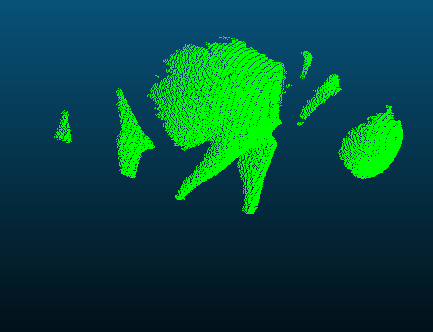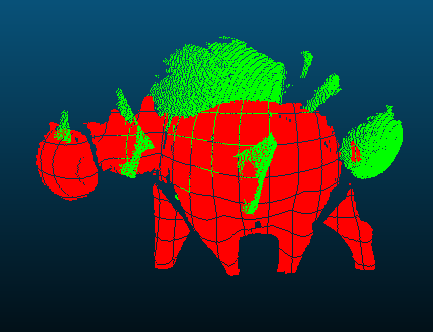Registered using OpenGR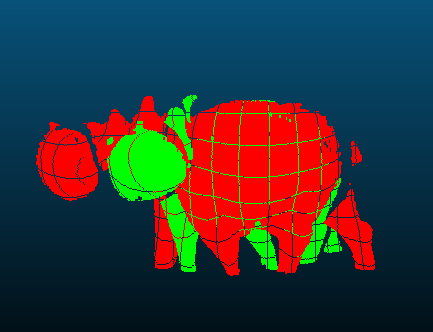Registered using PointMatcher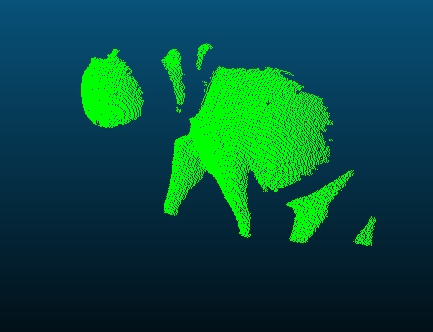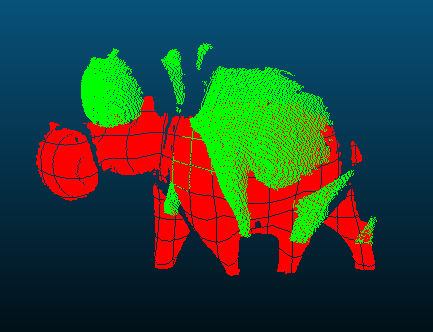Registered using OpenGR+PointMatcher Pipeline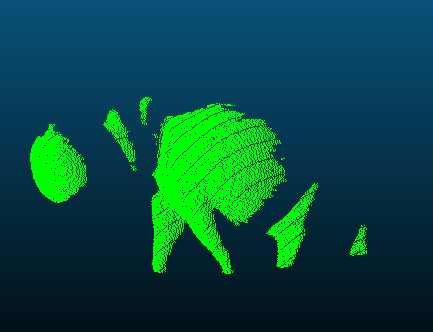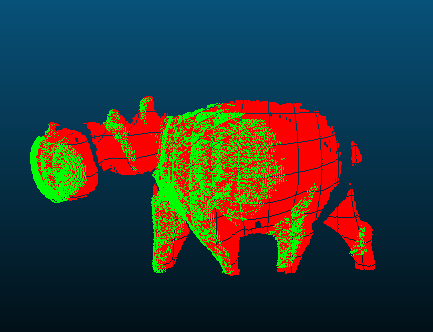Figure 79.4 Visualization of registered hippo scans with different registration methods. Two scans are used: red as the reference, green as the one for which the transformation is computed and applied. To obtain the results, the example code given in OpenGR Example , PointMatcher Example , OpenGR/PointMatcher Pipeline Example were applied, respectively. The parameters of the algorithms used to obtain those results are not optimized for the shown scans; therefore, better parameter choice might result in better results in terms of registration accuracy for each algorithm individually.

# Clustering

If an input point set represents several objects which are spatially separated, a clustering algorithm can be applied to identify connected components on a nearest neighbor graph built using a query sphere of fixed radius centered on each point.

The clustering is stored in a cluster map which associates each input point with the index of the cluster it belongs to: users can then use this map however they find it relevant to their use case, for example segmenting the input point set into one point set per cluster. Figure 79.13 shows different clustering outputs.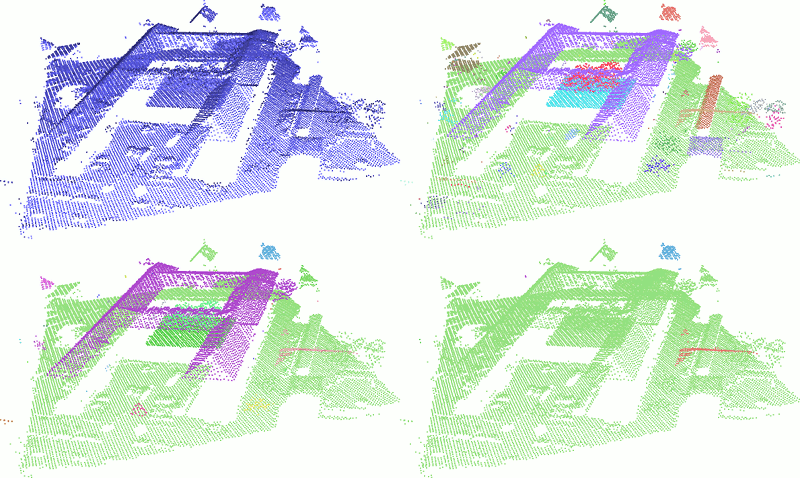Figure 79.13 Point Set Clustering outputs (one color per cluster). Top: input point set and clustering using a neighbor radius of 1.5 (147 clusters extracted). Bottom: clustering with neighbor radius 3.0 (37 clusters extracted), and with neighbor radius 6.0 (5 clusters extracted).

## Example

In the following example, clusters (and adjacencies between them) are computed and stored as colors in a PLY file:

#include <CGAL/Exact_predicates_inexact_constructions_kernel.h>
#include <CGAL/Point_set_3.h>
#include <CGAL/Point_set_3/IO.h>
#include <CGAL/cluster_point_set.h>
#include <CGAL/compute_average_spacing.h>
#include <CGAL/Random.h>
#include <CGAL/Real_timer.h>
#include <fstream>
#include <iostream>
using Point_3 = Kernel::Point_3;
using Point_set = CGAL::Point_set_3<Point_3>;
int main (int argc, char** argv)
{
std::ifstream ifile((argc > 1) ? argv : CGAL::data_file_path("points_3/hippo1.ply"), std::ios_base::binary);
Point_set points;
ifile >> points;
// Compute average spacing
double spacing = CGAL::compute_average_spacing<CGAL::Parallel_if_available_tag> (points, 12);
std::cerr << "Spacing = " << spacing << std::endl;
// Compute clusters
CGAL::Real_timer t;
t.start();
std::size_t nb_clusters
= CGAL::cluster_point_set(points, cluster_map,
t.stop();
std::cerr << "Found " << nb_clusters << " clusters with " << adjacencies.size()
<< " adjacencies in " << t.time() << " seconds" << std::endl;
// Output a colored PLY file
Point_set::Property_map<unsigned char> red = points.add_property_map<unsigned char>("red", 0).first;
Point_set::Property_map<unsigned char> green = points.add_property_map<unsigned char>("green", 0).first;
Point_set::Property_map<unsigned char> blue = points.add_property_map<unsigned char>("blue", 0).first;
for(Point_set::Index idx : points)
{
// One color per cluster
CGAL::Random rand (cluster_map[idx]);
red[idx] = rand.get_int(64, 192);
green[idx] = rand.get_int(64, 192);
blue[idx] = rand.get_int(64, 192);
}
std::ofstream ofile("out.ply", std::ios_base::binary);
ofile << points;
return EXIT_SUCCESS;
}

# Outlier Removal

Function remove_outliers() deletes a user-specified fraction of outliers from an input point set. More specifically, it partitions the input points with respect to the average squared distances to their nearest neighbors and deletes the points with largest value, either partitioning with a threshold or removing a fixed percentage. The user can either specify a fixed number of nearest neighbors or a fixed spherical neighborhood radius.

## Example

The following example reads a point set and removes 5% of the points. It uses the Identity_property_map<Point_3> property map (optional as it is the default position property map of all functions in this component.)
File Point_set_processing_3/remove_outliers_example.cpp

#include <CGAL/Exact_predicates_inexact_constructions_kernel.h>
#include <CGAL/property_map.h>
#include <CGAL/compute_average_spacing.h>
#include <CGAL/remove_outliers.h>
#include <vector>
#include <fstream>
#include <iostream>
// types
typedef Kernel::Point_3 Point;
int main(int argc, char*argv[])
{
const std::string fname = (argc>1)?argv:CGAL::data_file_path("points_3/oni.pwn");
// Reads a point set file in points[].
// The Identity_property_map property map can be omitted here as it is the default value.
std::vector<Point> points;
CGAL::parameters::point_map(CGAL::Identity_property_map<Point>())))
{
std::cerr << "Error: cannot read file " << fname << std::endl;
return EXIT_FAILURE;
}
// Removes outliers using erase-remove idiom.
// The Identity_property_map property map can be omitted here as it is the default value.
const int nb_neighbors = 24; // considers 24 nearest neighbor points
// Estimate scale of the point set with average spacing
const double average_spacing = CGAL::compute_average_spacing<CGAL::Sequential_tag>(points, nb_neighbors);
// FIRST OPTION //
// I don't know the ratio of outliers present in the point set
std::vector<Point>::iterator first_to_remove
= CGAL::remove_outliers<CGAL::Parallel_if_available_tag>
(points,
nb_neighbors,
CGAL::parameters::threshold_percent (100.). // No limit on the number of outliers to remove
threshold_distance (2. * average_spacing)); // Point with distance above 2*average_spacing are considered outliers
std::cerr << (100. * std::distance(first_to_remove, points.end()) / static_cast<double>(points.size()))
<< "% of the points are considered outliers when using a distance threshold of "
<< 2. * average_spacing << std::endl;
// SECOND OPTION //
// I know the ratio of outliers present in the point set
const double removed_percentage = 5.0; // percentage of points to remove
points.erase(CGAL::remove_outliers<CGAL::Parallel_if_available_tag>
(points,
nb_neighbors,
CGAL::parameters::threshold_percent(removed_percentage). // Minimum percentage to remove
threshold_distance(0.)), // No distance threshold (can be omitted)
points.end());
// Optional: after erase(), use Scott Meyer's "swap trick" to trim excess capacity
std::vector<Point>(points).swap(points);
return EXIT_SUCCESS;
}

# Simplification

Four simplification functions are devised to reduce an input point set.

Function random_simplify_point_set() randomly deletes a user-specified fraction of points from the input point set. This algorithm is the fastest.

Function grid_simplify_point_set() considers a regular grid covering the bounding box of the input point set, and clusters all points sharing the same cell of the grid by picking as representative one arbitrarily chosen point: representatives may only be retained in cells that have more than a user-defined minimum number of points in order to also filter out low density areas and outliers. This algorithm is still fast, although slower than random_simplify_point_set().

Function hierarchy_simplify_point_set() provides an adaptive simplification of the point set through local clusters . The size of the clusters is either directly selected by the user or it automatically adapts to the local variation of the point set.

Function wlop_simplify_and_regularize_point_set() not only simplifies, but also regularizes downsampled points. This is an implementation of the Weighted Locally Optimal Projection (WLOP) algorithm .

## Grid Simplification Example

The following example reads a point set and simplifies it by clustering. To filter out outliers, at least 3 points must lie in a cell so that a point from this cell is kept.
File Point_set_processing_3/grid_simplification_example.cpp

#include <CGAL/Exact_predicates_inexact_constructions_kernel.h>
#include <CGAL/grid_simplify_point_set.h>
#include <vector>
#include <fstream>
// types
typedef Kernel::Point_3 Point;
int main(int argc, char*argv[])
{
const std::string fname = (argc>1) ? argv : CGAL::data_file_path("points_3/oni.pwn");
// Reads a point set file in points[].
std::vector<Point> points;
{
std::cerr << "Error: cannot read file " << fname << std::endl;
return EXIT_FAILURE;
}
// simplification by clustering using erase-remove idiom
double cell_size = 0.03;
unsigned int min_points_per_cell = 3;
auto iterator_to_first_to_remove
(points, cell_size,
CGAL::parameters::min_points_per_cell(min_points_per_cell)); // optional
points.erase(iterator_to_first_to_remove, points.end());
// Optional: after erase(), shrink_to_fit to trim excess capacity
points.shrink_to_fit();
return EXIT_SUCCESS;
}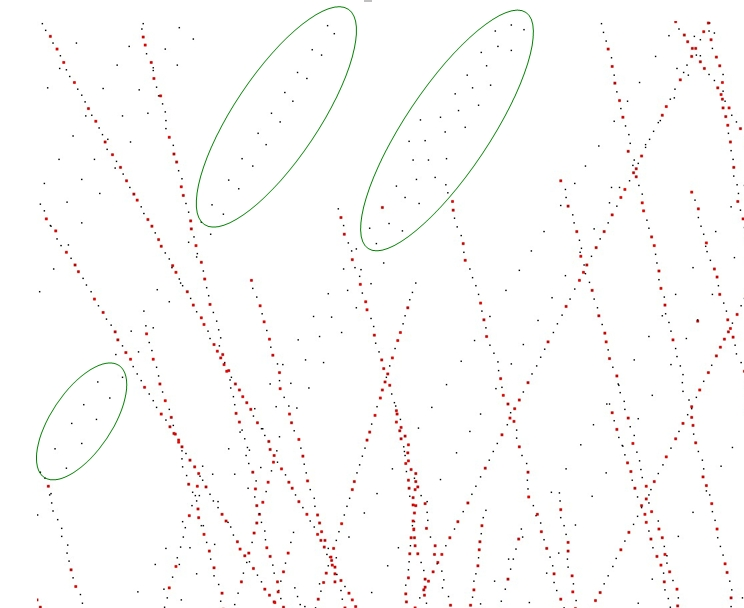Figure 79.14 Point set simplification through grid-based clustering. Removed points are depicted in red. Notice how low-density areas (in green) are not simplified.

## Hierarchy Simplification Example

The following example reads a point set and produces a set of clusters.

#include <CGAL/Exact_predicates_inexact_constructions_kernel.h>
#include <CGAL/IO/write_points.h>
#include <CGAL/hierarchy_simplify_point_set.h>
#include <CGAL/Memory_sizer.h>
#include <CGAL/Timer.h>
#include <vector>
#include <fstream>
// types
typedef Kernel::Point_3 Point;
int main(int argc, char*argv[])
{
const std::string fname = (argc>1) ? argv : CGAL::data_file_path("points_3/oni.pwn");
// Reads a point set file in points[].
std::vector<Point> points;
{
std::cerr << "Error: cannot read file " << fname << std::endl;
return EXIT_FAILURE;
}
std::cout << "Read " << points.size () << " point(s)" << std::endl;
// simplification by clustering using erase-remove idiom
CGAL::parameters::size(100)// Max cluster size
.maximum_variation(0.01)), // Max surface variation
points.end());
std::size_t memory = CGAL::Memory_sizer().virtual_size();
std::cout << points.size () << " point(s) kept, computed in "
<< task_timer.time() << " seconds, "
<< (memory>>20) << " Mib allocated." << std::endl;
CGAL::IO::write_points("out.xyz", points, CGAL::parameters::stream_precision(17));
return EXIT_SUCCESS;
}

### Parameter: size

The hierarchy simplification algorithm recursively split the point set in two until each cluster's size is less than the parameter size.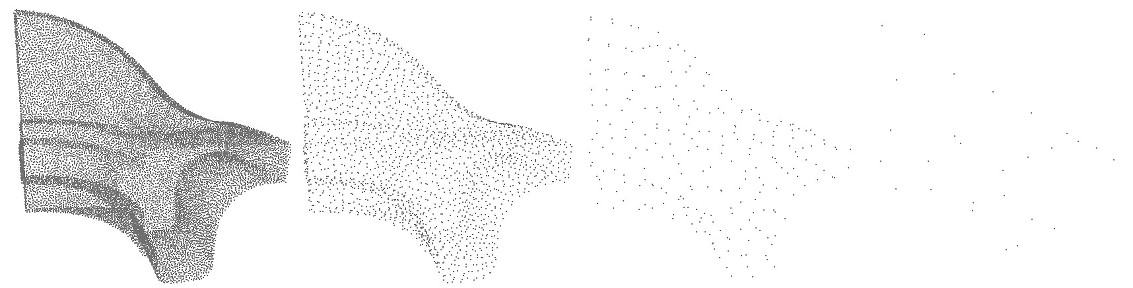Figure 79.15 Input point set and hierarchy simplification with different size parameter: $$10$$, $$100$$ and $$1000$$. In the 3 cases, var_max $$=1/3$$.

### Parameter: var_max

In addition to the size parameter, a variation parameter allows to increase simplification in monotonous regions. For each cluster, a surface variation measure is computed using the sorted eigenvalues of the covariance matrix:

$\sigma(p) = \frac{\lambda_0}{\lambda_0 + \lambda_1 + \lambda_2}.$

This function goes from $$0$$ if the cluster is coplanar to $$1/3$$ if it is fully isotropic. If a cluster's variation is above var_max, it is split. If var_max is equal to $$1/3$$, this parameter has no effect and the clustering is regular on the whole point set.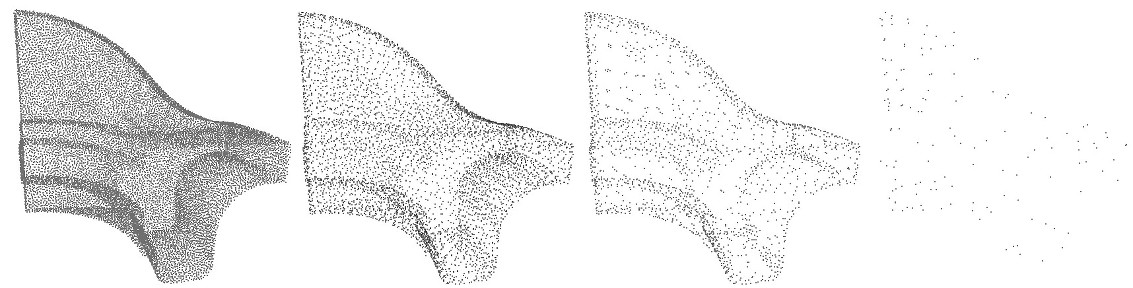Figure 79.16 Input point set and hierarchy simplification with different var_max parameter: $$0.00001$$, $$0.001$$ and $$0.1$$. In the 3 cases, size $$=1000$$.

## WLOP Simplification Example

The following example reads a point set, simplifies and regularizes it by WLOP.

#include <CGAL/Simple_cartesian.h>
#include <CGAL/wlop_simplify_and_regularize_point_set.h>
#include <CGAL/IO/write_points.h>
#include <vector>
#include <fstream>
#include <iostream>
// types
typedef Kernel::Point_3 Point;
// Concurrency
typedef CGAL::Parallel_if_available_tag Concurrency_tag;
int main(int argc, char** argv)
{
const std::string input_filename = (argc>1)?argv:CGAL::data_file_path("points_3/sphere_20k.xyz");
const char* output_filename = (argc>2)?argv:"data/sphere_20k_WLOPED.xyz";
// Reads a .xyz point set file in points[]
std::vector<Point> points;
{
std::cerr << "Error: cannot read file " << input_filename << std::endl;
return EXIT_FAILURE;
}
std::vector<Point> output;
//parameters
const double retain_percentage = 2; // percentage of points to retain.
const double neighbor_radius = 0.5; // neighbors size.
CGAL::wlop_simplify_and_regularize_point_set<Concurrency_tag>
(points, std::back_inserter(output),
CGAL::parameters::select_percentage(retain_percentage).
if(!CGAL::IO::write_points(output_filename, output, CGAL::parameters::stream_precision(17)))
return EXIT_FAILURE;
return EXIT_SUCCESS;
}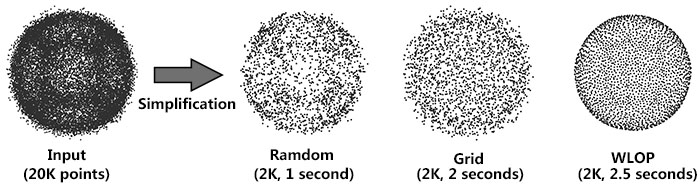Figure 79.17 Comparison for three simplification methods: Left: Random simplification result. Middle: Grid simplification result. Right: WLOP simplification result.

### Parameter: require_uniform_sampling

Computing density weights for each point is an optional preprocessing. For example, as shown in the following figure, when require_uniform_sampling is set to false, WLOP preserves the intrinsic non-uniform sampling of the original points; if require_uniform_sampling is set to true, WLOP is resilient to non-uniform sampling and generates sample points with more uniform distribution, at the expense of computational time.Figure 79.18 Comparison between with and without density: Left: input. Middle: require_uniform_sampling = false. Right: require_uniform_sampling=true.

Usually, the neighborhood of sample points should include at least two rings of neighboring sample points. Using a small neighborhood size may not be able to generate regularized result, while using big neighborhood size will make the sample points shrink into the interior of the local surface (under-fitting). The function will use a neighborhood size estimation if this parameter value is set to default or smaller that zero.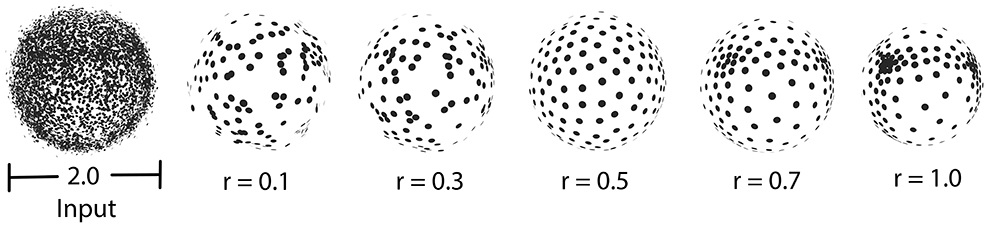Figure 79.19 Comparison between different sizes of neighbor radius.

### Parallel Performance

A parallel version of WLOP is provided and requires the executable to be linked against the Intel TBB library. To control the number of threads used, the user may use the tbb::task_scheduler_init class. See the TBB documentation for more details. We provide below a speed-up chart generated using the parallel version of the WLOP algorithm. The machine used is a PC running Windows 7 64-bits with a 4-core i7-47.nosp@m.00HQ.nosp@m.@2.40.nosp@m.GHz CPU with 8GB of RAM.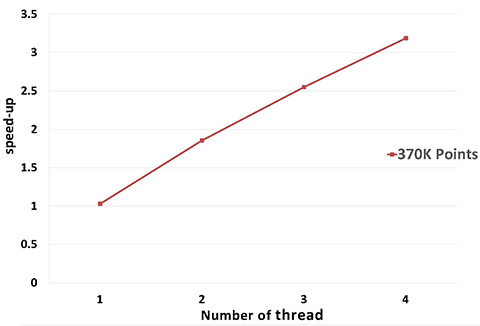Figure 79.20 Parallel WLOP speed-up, compared to the sequential version of the algorithm.

# Smoothing

Two smoothing functions are devised to smooth an input point set.

Function jet_smooth_point_set() smooths the input point set by projecting each point onto a smooth parametric surface patch (so-called jet surface) fitted over its nearest neighbors.

Function bilateral_smooth_point_set() smooths the input point set by iteratively projecting each point onto the implicit surface patch fitted over its nearest neighbors. Bilateral projection preserves sharp features according to the normal (gradient) information. Normals are thus required as input. For more details, see section 4 of .

For both functions, the user can either specify a fixed number of nearest neighbors or a fixed spherical neighborhood radius.

## Jet Smoothing Example

The following example generates a set of 9 points close to the xy plane and smooths them using 8 nearest neighbors:
File Point_set_processing_3/jet_smoothing_example.cpp

#include <CGAL/Exact_predicates_inexact_constructions_kernel.h>
#include <CGAL/jet_smooth_point_set.h>
#include <vector>
// types
typedef Kernel::Point_3 Point;
// Concurrency
typedef CGAL::Parallel_if_available_tag Concurrency_tag;
int main(void)
{
// generate point set
std::vector<Point> points;
points.push_back(Point( 0.0, 0.0, 0.001));
points.push_back(Point(-0.1,-0.1, 0.002));
points.push_back(Point(-0.1,-0.2, 0.001));
points.push_back(Point(-0.1, 0.1, 0.002));
points.push_back(Point( 0.1,-0.1, 0.000));
points.push_back(Point( 0.1, 0.2, 0.001));
points.push_back(Point( 0.2, 0.0, 0.002));
points.push_back(Point( 0.2, 0.1, 0.000));
points.push_back(Point( 0.0,-0.1, 0.001));
// Smoothing.
const unsigned int nb_neighbors = 8; // default is 24 for real-life point sets
CGAL::jet_smooth_point_set<Concurrency_tag>(points, nb_neighbors);
return EXIT_SUCCESS;
}

## Bilateral Smoothing Example

The following example reads a set of points with normals and smooths them via bilateral smoothing:
File Point_set_processing_3/bilateral_smooth_point_set_example.cpp

#include <CGAL/Simple_cartesian.h>
#include <CGAL/bilateral_smooth_point_set.h>
#include <CGAL/IO/write_points.h>
#include <CGAL/property_map.h>
#include <CGAL/tags.h>
#include <utility> // defines std::pair
#include <fstream>
// Types
typedef Kernel::Point_3 Point;
typedef Kernel::Vector_3 Vector;
// Point with normal vector stored in a std::pair.
typedef std::pair<Point, Vector> PointVectorPair;
// Concurrency
typedef CGAL::Parallel_if_available_tag Concurrency_tag;
int main(int argc, char*argv[])
{
const std::string input_filename = (argc>1) ? argv : CGAL::data_file_path("points_3/fin90_with_PCA_normals.xyz");
const char* output_filename = (argc>2) ? argv : "data/fin90_with_PCA_normals_bilateral_smoothed.xyz";
// Reads a point set file in points[] * with normals *.
std::vector<PointVectorPair> points;
{
std::cerr << "Error: cannot read file " << input_filename << std::endl;
return EXIT_FAILURE;
}
// Algorithm parameters
int k = 120; // size of neighborhood. The bigger the smoother the result will be.
// This value should bigger than 1.
double sharpness_angle = 25; // control sharpness of the result.
// The bigger the smoother the result will be
int iter_number = 3; // number of times the projection is applied
for(int i = 0; i < iter_number; ++i)
{
/* double error = */
CGAL::bilateral_smooth_point_set <Concurrency_tag>(
points,
k,
.sharpness_angle(sharpness_angle));
}
if(!CGAL::IO::write_XYZ(output_filename, points,
.stream_precision(17)))
return EXIT_FAILURE;
return EXIT_SUCCESS;
}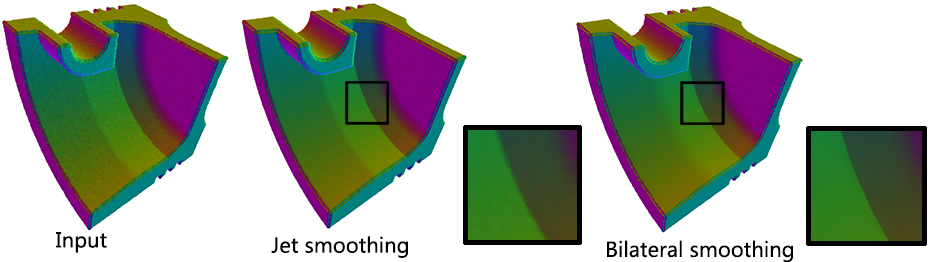Figure 79.21 Comparison for two smoothing methods: Left: Input, 250K points, normal-color mapping. Middle: Jet smoothing result, 197 seconds. Right: Bilateral smoothing result, 110 seconds.

### Parallel

Performance: A parallel version of bilateral smoothing is provided and requires the executable to be linked against the Intel TBB library. The number of threads used is controlled through the tbb::task_scheduler_init class. See the TBB documentation for more details. We provide below a speed-up chart generated using the parallel version of the bilateral smoothing algorithm. The machine used is a PC running Windows 7 64-bits with a 4-core i7-47.nosp@m.00HQ.nosp@m.@2.40.nosp@m.GHz CPU with 8GB of RAM.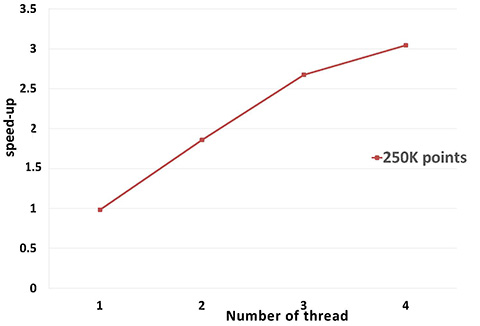Figure 79.22 Parallel bilateral smoothing speed-up, compared to the sequential version of the algorithm.

# Normal Estimation

Assuming a point set sampled over an inferred surface S, two functions provide an estimate of the normal to S at each point. The result is an unoriented normal vector for each input point.

Function jet_estimate_normals() estimates the normal direction at each point from the input set by fitting a jet surface over its nearest neighbors. The default jet is a quadric surface. This algorithm is well suited to point sets scattered over curved surfaces.

Function pca_estimate_normals() estimates the normal direction at each point from the set by linear least squares fitting of a plane over its nearest neighbors. This algorithm is simpler and faster than jet_estimate_normals().

Function vcm_estimate_normals() estimates the normal direction at each point from the set by using the Voronoi Covariance Measure of the point set. This algorithm is more complex and slower than the previous algorithms. It is based on the article .

For these three functions, the user can either specify a fixed number of nearest neighbors or a fixed spherical neighborhood radius.

# Normal Orientation

## Minimum Spanning Tree

Function mst_orient_normals() orients the normals of a set of points with unoriented normals using the method described by Hoppe et al. in Surface reconstruction from unorganized points . More specifically, this method constructs a Riemannian graph over the input points (the graph of the nearest neighbor points) and propagates a seed normal orientation within a minimum spanning tree computed over this graph. The result is an oriented normal vector for each input unoriented normal, except for the normals which cannot be successfully oriented.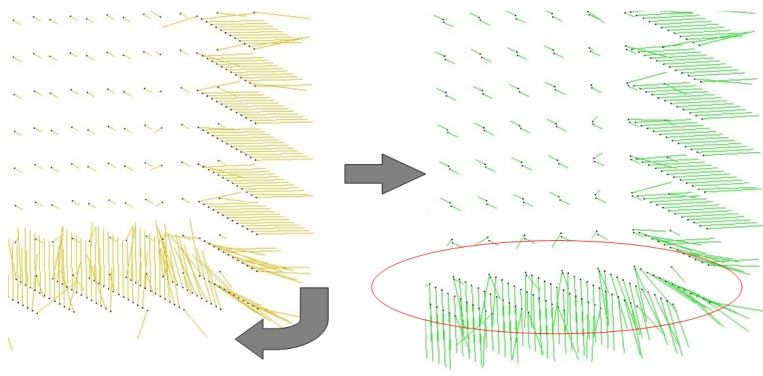Figure 79.23 Normal orientation of a sampled cube surface. Left: unoriented normals. Right: orientation of right face normals is propagated to bottom face.

### Example

The following example reads a point set from a file, estimates the normals through PCA (either over the 18 nearest neighbors or using a spherical neighborhood radius of twice the average spacing) and orients the normals:
File Point_set_processing_3/normals_example.cpp

#include <CGAL/Exact_predicates_inexact_constructions_kernel.h>
#include <CGAL/compute_average_spacing.h>
#include <CGAL/pca_estimate_normals.h>
#include <CGAL/mst_orient_normals.h>
#include <CGAL/property_map.h>
#include <utility> // defines std::pair
#include <list>
#include <fstream>
// Types
typedef Kernel::Point_3 Point;
typedef Kernel::Vector_3 Vector;
// Point with normal vector stored in a std::pair.
typedef std::pair<Point, Vector> PointVectorPair;
// Concurrency
typedef CGAL::Parallel_if_available_tag Concurrency_tag;
int main(int argc, char*argv[])
{
const std::string fname = (argc>1) ? argv : CGAL::data_file_path("points_3/sphere_1k.xyz");
// Reads a point set file in points[].
std::list<PointVectorPair> points;
std::back_inserter(points),
CGAL::parameters::point_map(CGAL::First_of_pair_property_map<PointVectorPair>())))
{
std::cerr << "Error: cannot read file " << fname<< std::endl;
return EXIT_FAILURE;
}
// Estimates normals direction.
// Note: pca_estimate_normals() requiresa range of points
// as well as property maps to access each point's position and normal.
const int nb_neighbors = 18; // K-nearest neighbors = 3 rings
if (argc > 2 && std::strcmp(argv, "-r") == 0) // Use a fixed neighborhood radius
{
// First compute a spacing using the K parameter
double spacing
= CGAL::compute_average_spacing<Concurrency_tag>
(points, nb_neighbors,
CGAL::parameters::point_map(CGAL::First_of_pair_property_map<PointVectorPair>()));
// Then, estimate normals with a fixed radius
CGAL::pca_estimate_normals<Concurrency_tag>
(points,
0, // when using a neighborhood radius, K=0 means no limit on the number of neighbors returns
}
else // Use a fixed number of neighbors
{
CGAL::pca_estimate_normals<Concurrency_tag>
(points, nb_neighbors,
}
// Orients normals.
// Note: mst_orient_normals() requires a range of points
// as well as property maps to access each point's position and normal.
std::list<PointVectorPair>::iterator unoriented_points_begin =
CGAL::mst_orient_normals(points, nb_neighbors,
// Optional: delete points with an unoriented normal
// if you plan to call a reconstruction algorithm that expects oriented normals.
points.erase(unoriented_points_begin, points.end());
return EXIT_SUCCESS;
}

## Scanline

The minimum spanning tree results can give suboptimal results on point clouds with many sharp features and occlusions, which typically happens on airborne acquired urban datasets.

scanline_orient_normals() is an alternative method specialized for point sets which are ordered in scanline aligned on the XY-plane. It can take advantage of LAS properties provided by some LIDAR scanner and is the best choice of normal orientation when dealing with 2.5D urban scenes.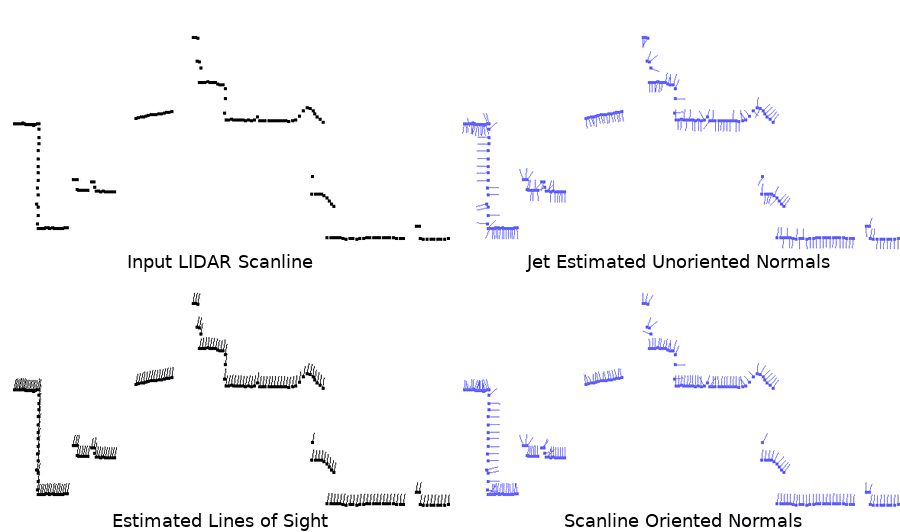Figure 79.24 Normal orientation of a LIDAR scanline. The point cloud is a typical airborne LIDAR input, sampling a building without normal information and with many occlusions (especially on vertical walls).

### Example

The following example reads a point set from a LAS file, estimates the normals through Jet Fitting and outputs in PLY format the orientation results of all the variants of scanline_orient_normals():

#include <CGAL/Simple_cartesian.h>
#include <CGAL/IO/write_ply_points.h>
#include <CGAL/jet_estimate_normals.h>
#include <CGAL/scanline_orient_normals.h>
using Point_3 = Kernel::Point_3;
using Vector_3 = Kernel::Vector_3;
using Point_with_info = std::tuple<Point_3, Vector_3, float, unsigned char>;
void dump (const char* filename, const std::vector<Point_with_info>& points)
{
std::ofstream ofile (filename, std::ios::binary);
(ofile, points,
CGAL::parameters::point_map (Point_map()).
normal_map (Normal_map()));
}
int main (int argc, char** argv)
{
std::string fname (argc > 1 ? argv : "data/urban.las");
std::vector<Point_with_info> points;
std::cerr << "Reading input file " << fname << std::endl;
std::ifstream ifile (fname, std::ios::binary);
if (!ifile ||
(ifile, std::back_inserter (points),
std::make_pair (Scan_angle_map(),
CGAL::IO::LAS_property::Scan_angle()),
std::make_pair (Scanline_id_map(),
CGAL::IO::LAS_property::Scan_direction_flag())))
{
std::cerr << "Can't read " << fname << std::endl;
return EXIT_FAILURE;
}
std::cerr << "Estimating normals" << std::endl;
CGAL::jet_estimate_normals<CGAL::Parallel_if_available_tag>
(points, 12,
CGAL::parameters::point_map (Point_map()).
normal_map (Normal_map()));
std::cerr << "Orienting normals using scan angle and direction flag" << std::endl;
(points,
CGAL::parameters::point_map (Point_map()).
normal_map (Normal_map()).
scan_angle_map (Scan_angle_map()).
scanline_id_map (Scanline_id_map()));
dump("out_angle_and_flag.ply", points);
std::cerr << "Orienting normals using scan direction flag only" << std::endl;
(points,
CGAL::parameters::point_map (Point_map()).
normal_map (Normal_map()).
scanline_id_map (Scanline_id_map()));
dump("out_flag.ply", points);
std::cerr << "Orienting normals using scan angle only" << std::endl;
(points,
CGAL::parameters::point_map (Point_map()).
normal_map (Normal_map()).
scan_angle_map (Scan_angle_map()));
dump("out_angle.ply", points);
std::cerr << "Orienting normals using no additional info" << std::endl;
(points,
CGAL::parameters::point_map (Point_map()).
normal_map (Normal_map()));
dump("out_nothing.ply", points);
return EXIT_SUCCESS;
}

# Upsampling

The function edge_aware_upsample_point_set() generates a denser point set from an input point set. This has applications in point-based rendering, hole filling, and sparse surface reconstruction. The algorithm can progressively upsample the point set while approaching the edge singularities. See  for more details.

## Example

The following example reads a point set from a file, upsamples it to get a denser result.

#include <CGAL/Simple_cartesian.h>
#include <CGAL/edge_aware_upsample_point_set.h>
#include <CGAL/IO/write_points.h>
#include <vector>
#include <fstream>
// types
typedef Kernel::Point_3 Point;
typedef Kernel::Vector_3 Vector;
// Point with normal vector stored in a std::pair.
typedef std::pair<Point, Vector> PointVectorPair;
// Concurrency
typedef CGAL::Parallel_if_available_tag Concurrency_tag;
int main(int argc, char* argv[])
{
const std::string input_filename = (argc>1) ? argv : CGAL::data_file_path("points_3/before_upsample.xyz");
const char* output_filename = (argc>2) ? argv : "data/before_upsample_UPSAMPLED.xyz";
// Reads a .xyz point set file in points[], *with normals*.
std::vector<PointVectorPair> points;
std::back_inserter(points),
{
std::cerr << "Error: cannot read file " << input_filename << std::endl;
return EXIT_FAILURE;
}
//Algorithm parameters
const double sharpness_angle = 25; // control sharpness of the result.
const double edge_sensitivity = 0; // higher values will sample more points near the edges
const double neighbor_radius = 0.25; // initial size of neighborhood.
const std::size_t number_of_output_points = points.size() * 4;
//Run algorithm
CGAL::edge_aware_upsample_point_set<Concurrency_tag>(
points,
std::back_inserter(points),
CGAL::parameters::point_map(CGAL::First_of_pair_property_map<PointVectorPair>()).
sharpness_angle(sharpness_angle).
edge_sensitivity(edge_sensitivity).
number_of_output_points(number_of_output_points));
// Saves point set.
if(!CGAL::IO::write_points(output_filename, points,
.stream_precision(17)))
return EXIT_FAILURE;
return EXIT_SUCCESS;
}

### Parameter: edge_sensitivity

This parameter controls where the new points are inserted. Larger values of edge-sensitivity give higher priority to inserting points along the sharp features. For example, as shown in the following figure, high value is preferable when one wants to insert more points on sharp features, where the local gradient is high, e.g., darts, cusps, creases and corners. In contrast, points are evenly inserted when edge_sensitivity is set to 0. The range of possible value is [0, 1].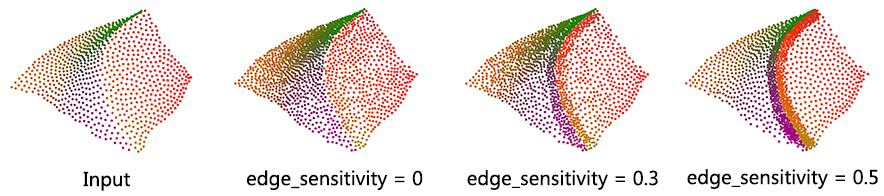Figure 79.25 Upsampling for different edge-sensitivity parameter values. The input containing 850 points is upsampled to 1,500 points in all cases depicted.

### Parameter: sharpness_angle

This parameter controls the preservation of sharp features.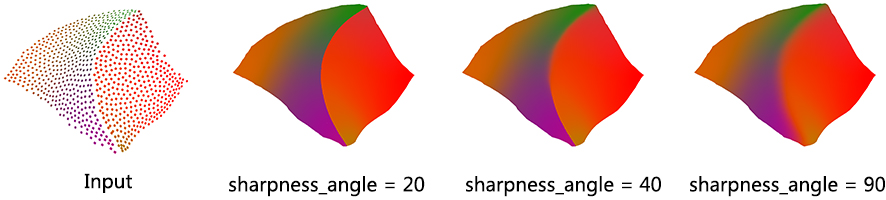Figure 79.26 Upsampling for different sharpness_angle parameter values. The input containing 850 points is upsampled to 425K points in all cases depicted.

Usually, the neighborhood of sample points should include at least one ring of neighboring sample points. Using small neighborhood size may not be able to insert new points. Using big neighborhood size can fill small holes, but points inserted on the edges could be irregular. The function will use a neighborhood size estimation if this parameter value is set to default or smaller than zero.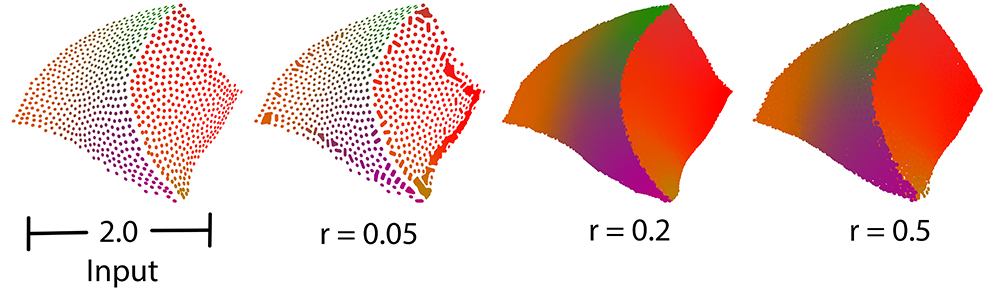Figure 79.27 Comparison between different sizes of neighbor radius.

# Feature Edges Estimation

Function vcm_is_on_feature_edge() indicates if a points belong to a feature edges of the point set using its Voronoi Covariance Measure. It is based on the article .

It first computes the VCM of the points set using compute_vcm(). Then, it estimates which points belong to a sharp edge by testing if a ratio of eigenvalues is greater than a given threshold.

## Example

The following example reads a point set from a file, estimates the points that are on sharp edges:
File Point_set_processing_3/edges_example.cpp

#include <CGAL/Exact_predicates_inexact_constructions_kernel.h>
#include <CGAL/vcm_estimate_edges.h>
#include <CGAL/property_map.h>
#include <utility> // defines std::pair
#include <vector>
#include <fstream>
// Types
typedef Kernel::Point_3 Point;
typedef Kernel::Vector_3 Vector;
// Point with normal vector stored in a std::pair.
typedef std::pair<Point, Vector> PointVectorPair;
typedef std::vector<PointVectorPair> PointList;
typedef std::array<double,6> Covariance;
int main (int , char**)
{
// Reads a polygon mesh file in points[].
std::list<PointVectorPair> points;
std::back_inserter(points),
CGAL::parameters::point_map(CGAL::First_of_pair_property_map<PointVectorPair>())))
{
std::cerr << "Error: cannot read file data/fandisk_large.off" << std::endl;
return EXIT_FAILURE;
}
// Estimates covariance matrices per points.
double R = 0.2,
r = 0.1;
std::vector<Covariance> cov;
CGAL::compute_vcm(points, cov, R, r,
CGAL::parameters::point_map (point_map).geom_traits (Kernel()));
// Find the points on the edges.
// Note that this step is not expensive and can be done several time to get better results
double threshold = 0.16;
std::ofstream output("points_on_edges.xyz");
int i = 0;
for(const PointVectorPair& p : points)
{
if(CGAL::vcm_is_on_feature_edge(cov[i], threshold))
output << p.first << "\n";
++i;
}
return 0;
}

# Structuring

The function structure_point_set() generates a structured version of the input point set assigned to a set of planes. Such an input can be produced by a shape detection algorithm (see Shape Detection Reference). Point set structuring is based on the article .

• Planes: inliers of each detected plane are replaced by sets of noise-free points sampled at the vertices of a regular grid: this is achieved by filling an occupancy grid aligned on the principal components of the inlier sets with a spacing lower than $$\sqrt{2}$$ times the user-defined tolerance.
• Creases: adjacencies between 2 planes are detected and regularly resampled on an occupancy array along the edge with a spacing equal to twice the user-defined tolerance.
• Corners: 3-cycles are detected from the primitive adjacency graph and sampled using the exact intersection point of the 3 planes (provided it exists and remains in the given tolerance). These corners are also locally clustered to former corners of degree higher than 3.

This algorithm is well suited to point sets sampled on surfaces with planar sections and sharp edges.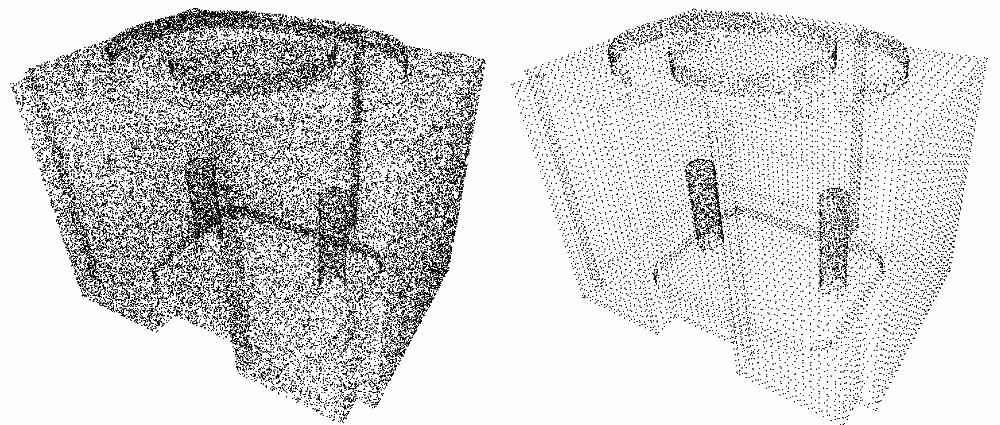Figure 79.28 Point set structuring. Left: input raw point set. Right: structured point set.

Structure information of points can be used to perform feature preserving reconstruction (see Advancing Front Surface Reconstruction for example). More specifically, the class storing a point set with structure provides the user with a method Point_set_with_structure::facet_coherence() that estimates if a triplet of points form a coherent facet.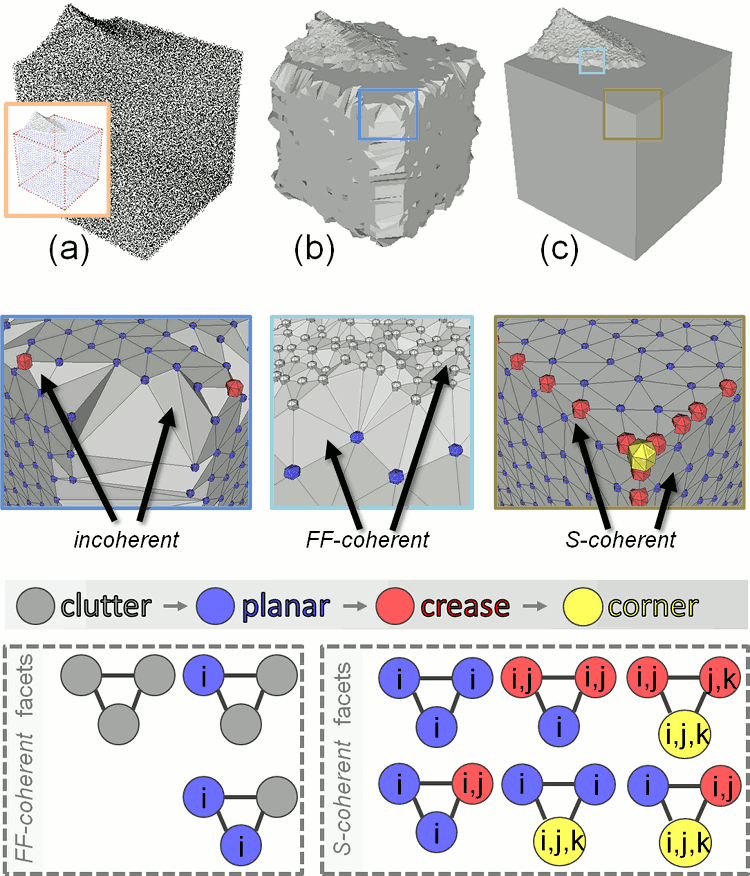Figure 79.29 (a) Input point set (and structured output); (b) output with many incoherent facets; (c) output with all facets coherent. i, j and k each corresponds to a primitive index.

## Example

The following example applies shape detection followed by structuring to a point set:
File Point_set_processing_3/structuring_example.cpp

#include <CGAL/Exact_predicates_inexact_constructions_kernel.h>
#include <CGAL/IO/write_points.h>
#include <CGAL/property_map.h>
#include <CGAL/Shape_detection/Efficient_RANSAC.h>
#include <CGAL/structure_point_set.h>
#include <iostream>
#include <fstream>
// Type declarations
typedef Kernel::Point_3 Point;
typedef std::pair<Kernel::Point_3, Kernel::Vector_3> Point_with_normal;
typedef std::vector<Point_with_normal> Pwn_vector;
// Efficient RANSAC types
<Kernel, Pwn_vector, Point_map, Normal_map> Traits;
int main (int argc, char** argv)
{
const std::string filename = (argc>1) ? argv : CGAL::data_file_path("points_3/cube.pwn");
// Points with normals.
Pwn_vector points;
CGAL::parameters::point_map(Point_map())
.normal_map(Normal_map())))
{
std::cerr << "Error: cannot read file cube.pwn" << std::endl;
return EXIT_FAILURE;
}
std::cerr << points.size() << " point(s) read." << std::endl;
// Shape detection
Efficient_ransac ransac;
ransac.set_input(points);
ransac.detect();
Efficient_ransac::Plane_range planes = ransac.planes();
Pwn_vector structured_pts;
planes,
std::back_inserter(structured_pts),
0.015, // epsilon for structuring points
CGAL::parameters::point_map(Point_map())
.normal_map(Normal_map())
.plane_index_map(CGAL::Shape_detection::Point_to_shape_index_map<Traits>(points, planes)));
std::cerr << structured_pts.size ()
<< " structured point(s) generated." << std::endl;
CGAL::IO::write_points("out.pwn", structured_pts,
CGAL::parameters::point_map(Point_map())
.normal_map(Normal_map())
.stream_precision(17));
return EXIT_SUCCESS;
}

# Callbacks

Several functions of this package provide a callback mechanism that enables the user to track the progress of the algorithms and to interrupt them if needed. A callback, in this package, is an instance of std::function<bool(double)> that takes the advancement as a parameter (between 0. when the algorithm begins to 1. when the algorithm is completed) and that returns true if the algorithm should carry on, false otherwise. It is passed as a named parameter with an empty function as default.

Algorithms that support this mechanism are detailed in the Reference Manual, along with the effect that an early interruption has on the output.

## Example

The following example defines a callback that displays the name of the current algorithm along with the progress (as a percentage) updated every $$1/10th$$ of a second. While the algorithm is running, the console output will typically look like this:

Computing average spacing: 100%
Grid simplification: 100%
Jet smoothing: 54%

Thanks to the carriage return character \r, the lines are overwritten and the user sees the percentage increasing on each line.

#include <CGAL/Exact_predicates_inexact_constructions_kernel.h>
#include <CGAL/point_generators_3.h>
#include <CGAL/Real_timer.h>
#include <CGAL/compute_average_spacing.h>
#include <CGAL/grid_simplify_point_set.h>
#include <CGAL/jet_smooth_point_set.h>
#include <boost/lexical_cast.hpp>
#include <vector>
#include <fstream>
// Types
typedef Kernel::FT FT;
typedef Kernel::Point_3 Point;
typedef CGAL::Random_points_on_sphere_3<Point> Generator;
// Concurrency
typedef CGAL::Parallel_if_available_tag Concurrency_tag;
// instance of std::function<bool(double)>
struct Progress_to_std_cerr_callback
{
mutable std::size_t nb;
CGAL::Real_timer timer;
double t_start;
mutable double t_latest;
const std::string name;
Progress_to_std_cerr_callback (const char* name)
: name (name)
{
timer.start();
t_start = timer.time();
t_latest = t_start;
}
{
// Avoid calling time() at every single iteration, which could
++ nb;
if (advancement != 1 && nb % 100 != 0)
return true;
double t = timer.time();
if (advancement == 1 || (t - t_latest) > 0.1) // Update every 1/10th of second
{
std::cerr << "\r" // Return at the beginning of same line and overwrite
<< name << ": " << int(advancement * 100) << "%";
std::cerr << std::endl;
t_latest = t;
}
return true;
}
};
int main (int argc, char* argv[])
{
int N = (argc > 1) ? boost::lexical_cast<int>(argv) : 1000;
// Generate N points on a sphere of radius 100.
std::vector<Point> points;
points.reserve(N);
Generator generator(100.);
std::copy_n(generator, N, std::back_inserter(points));
// Compute average spacing
FT average_spacing = CGAL::compute_average_spacing<Concurrency_tag>
(points, 6,
CGAL::parameters::callback(Progress_to_std_cerr_callback("Computing average spacing")));
// Simplify on a grid with a size of twice the average spacing
points.erase(CGAL::grid_simplify_point_set(points, 2. * average_spacing,
CGAL::parameters::callback(Progress_to_std_cerr_callback("Grid simplification"))),
points.end());
// Smooth simplified point set
CGAL::jet_smooth_point_set<Concurrency_tag>(points, 6,
CGAL::parameters::callback(Progress_to_std_cerr_callback("Jet smoothing")));
return EXIT_SUCCESS;
}

# Implementation History

Pierre Alliez and Laurent Saboret contributed the initial component. Nader Salman contributed the grid simplification. Started from GSoC'2013, three new algorithms were implemented by Shihao Wu and Clément Jamin: WLOP, bilateral smoothing and upsampling. Started from GSoC'2014, Jocelyn Meyron with the help of Quentin Mérigot introduced the computation of the Voronoi covariance measure of a point set, as well as the normal and feature edge estimation functions based on it. Florent Lafarge with the help of Simon Giraudot contributed the point set structuring algorithm. Started from GSoC'2019, Necip Fazil Yildiran with the help of Nicolas Mellado and Simon Giraudot introduced the wrappers for OpenGR and PointMatcher libraries that perform registration on two point sets.